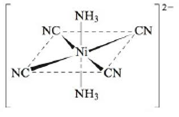# Sketch and explain the most likely crystal field diagram for the following complex ion: Note: The CN – ligand produces a much stronger crystal field than NH 3 . Assume the NH 3 ligands lie on the z axis.### Chemistry: An Atoms First Approach

2nd Edition
Steven S. Zumdahl + 1 other
Publisher: Cengage Learning
ISBN: 9781305079243

#### Solutions

Chapter
Section### Chemistry: An Atoms First Approach

2nd Edition
Steven S. Zumdahl + 1 other
Publisher: Cengage Learning
ISBN: 9781305079243
Chapter 20, Problem 97CP
Textbook Problem
1 views

## Sketch and explain the most likely crystal field diagram for the following complex ion:Note: The CN– ligand produces a much stronger crystal field than NH3. Assume the NH3 ligands lie on the z axis.

Interpretation Introduction

Interpretation: Considering NH3 ligands to lie on the z axis, crystal field splitting of the given complex ion is to be drawn.

Concept introduction: The electrons in the d orbital of a transition metal split into high and low energy orbitals when ligands are attached to it. The energy difference between these two levels depends upon the properties of both metal and the ligands. If the ligand is strong, then splitting will be high and the complex will be low spin. If the ligand is weak, then splitting will be less and the complex will be high spin.

To determine: The crystal field splitting diagram of the given complex.

### Explanation of Solution

Explanation

The given complex is,

Figure 1

The ligands CN- are in square planar arrangement. It is given that CN- ligands produces a much stronger crystal field than NH3 and NH3 ligands lie on z axis, therefore the crystal field diagram for this complex ion will resemble that of a square planar complex which is,

Figure 2

The CN- ligands are oriented along the x and y axes

### Still sussing out bartleby?

Check out a sample textbook solution.

See a sample solution

#### The Solution to Your Study Problems

Bartleby provides explanations to thousands of textbook problems written by our experts, many with advanced degrees!

Get Started

Find more solutions based on key concepts
A represents a quantitative observation.

Introductory Chemistry: A Foundation

A deficiency of which mineral is a leading cause of mental retardation worldwide? a. iron b. iodine c. zinc d. ...

Nutrition: Concepts and Controversies - Standalone book (MindTap Course List)

How do eating disorders affect health?

Understanding Nutrition (MindTap Course List)

Why are mathematical results rounded?

An Introduction to Physical Science

What does the word apparent mean in apparent visual magnitude?

Horizons: Exploring the Universe (MindTap Course List)

How is a population different from a community? A niche from a habitat?

Oceanography: An Invitation To Marine Science, Loose-leaf Versin

A conducting rod of length moves on two horizontal, frictionless rails as shown in Figure P31.26. If a constan...

Physics for Scientists and Engineers, Technology Update (No access codes included)

a 21.7 m to cm, b 517 m to km, c 0.666 km to cm

Introductory Chemistry: An Active Learning Approach